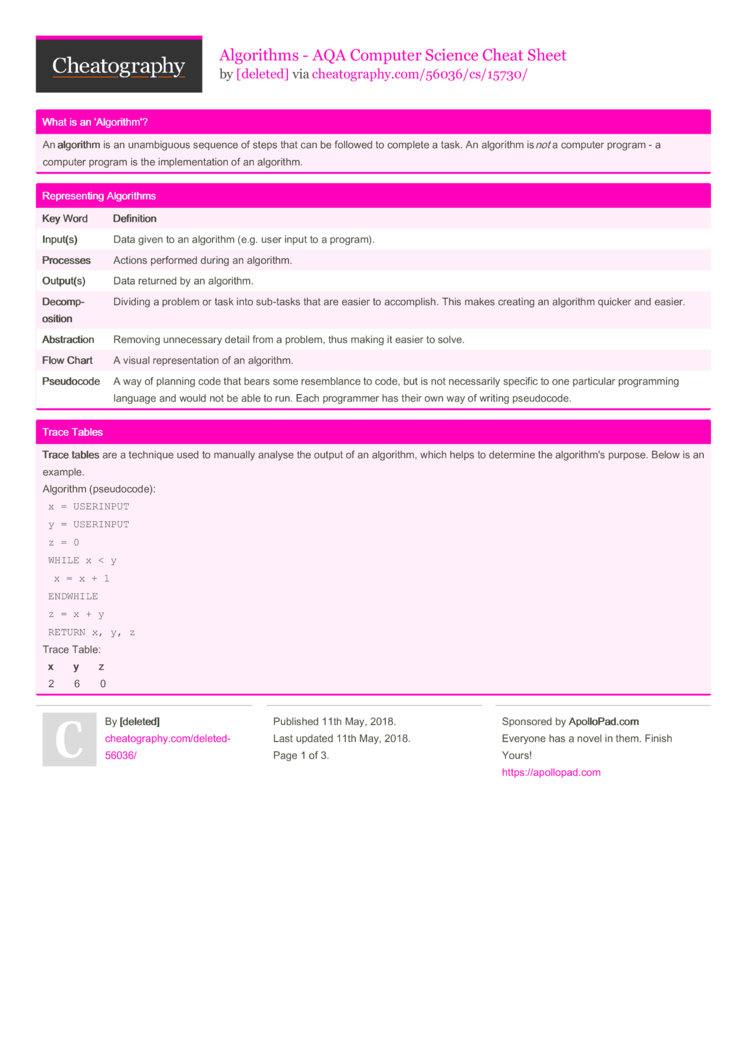# Algorithms - AQA Computer Science Cheat Sheet by [deleted]

### What is an 'Algor­ithm'?

 An algo­rithm is an unambi­guous sequence of steps that can be followed to complete a task. An algorithm is not a computer program - a computer program is the implem­ent­ation of an algorithm.

### Repres­enting Algorithms

 Key Word Defi­nit­ion Inpu­t(s) Data given to an algorithm (e.g. user input to a program). Proc­esses Actions performed during an algorithm. Outp­ut(s) Data returned by an algorithm. Deco­mpo­sit­ion Dividing a problem or task into sub-tasks that are easier to accomp­lish. This makes creating an algorithm quicker and easier. Abst­rac­tion Removing unnece­ssary detail from a problem, thus making it easier to solve. Flow Chart A visual repres­ent­ation of an algorithm. Pseu­doc­ode A way of planning code that bears some resemb­lance to code, but is not necess­arily specific to one particular progra­mming language and would not be able to run. Each programmer has their own way of writing pseudo­code.

### Trace Tables

 Trace tables are a technique used to manually analyse the output of an algorithm, which helps to determine the algori­thm's purpose. Below is an example. Algorithm (pseud­ocode):  ­ `x = USERINPUT`  ­ `y = USERINPUT`  ­ `z = 0`  ­ ­`WHILE x < y`  ­ ­ ­ `x = x + 1`  ­ ­`EN­DWHILE`  ­ `z = x + y`  ­ ­`RETURN x, y, z` Trace Table:   x­ ­ ­ ­ ­ ­ ­ ­y       z  ­ ­2       6       0  ­ ­3       6       0  ­ ­4       6       0  ­ ­5       6       0  ­ ­6       6       12

### Efficiency of Algorithms

 Generally, more than one algorithm can be used to solve a given problem. The one that is usually preferred is the most efficient one. Although there are multiple ways of measuring effici­ency, the time taken to execute a program is the main one. Obviously, a quicker solution is best (so that no time is wasted), so shorter algorithms are preferred.
For the AQA specif­ica­tion, "exam questions in this area will only refer to time effici­enc­y."

### Searching Algorithms

 Sear­ching algori­thms are procedures used to find a specific item of data in a dataset. A linear search begins at the start of the dataset, then moves through it sequen­tially, testing every element for a match. This means that it can be used on sorted or unsorted data and on an array of any data type. However, if the array is long then a linear search can be quite slow and take a long time. A binary search requires an orde­red data set, but is generally quic­ker than a linear search. It divides a list in half and 'looks at' the middle element (if there is an even number of elements, the first item on the right is examined). If the element is not equal to the desired value, then the algorithm checks whether the element is greater than the desired value. If so, the left-hand side of the list is discarded. If not, the right-hand side is discarded. This is repeated until the correct element is found. Both search algorithms cannot search for multiple occure­nces of an element in the same data set.

### Sorting Algorithms

 Sorting algori­thms are procedures used to arrange a data set into an order (usually ascend­ing). A bubble sort starts at the beginning of the data set. It then goes through it sequen­tially, checking each item against the next one. If the first item is greater than the second one, they are swapped. This is repeated until it reaches the end of the data set. It then makes a second pass, doing the same thing. Once it makes one full pass without making any changes, it terminates because the list is in order. A merge sort starts by dividing the list in half repeat­edly, until it is left with individual elements. It then merges these elements into pairs, with the smallest element on the left-hand side. This is repeated until all of the sublists are merged into one, which is now ordered. Bubble sorts are extr­emely ineffi­cie­nt, whereas merge sorts are very fast. However, merge sorts need addi­tional storage because there are many more stages.3 Pages
//media.cheatography.com/storage/thumb/deleted-56036_algorithms-aqa-computer-science.750.jpg

PDF (recommended)